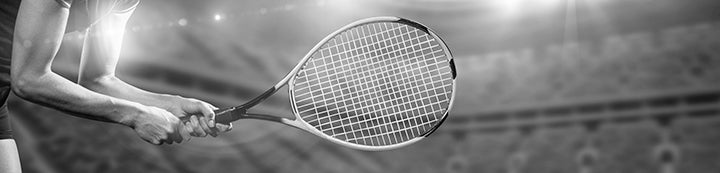21 - 30 of 30 Posts

#### silvamarius

·
##### Registered
Joined
·
1,928 Posts
Crawford d. Maria
Erakovic d. Loeb
Mattek-Sands d. Duque-Marino
Vickery d. Townsend
Cepede Royg d. Kostova

#### Tennisfan06

·
##### Registered
Joined
·
10,440 Posts
Re: Q-Ball ACAPULCO

Crawford d. Maria
Loeb d. Erakovic
Mattek-Sands d. Duque-Marino
Townsend d. Vickery
Cepede Royg d. Kostova

#### .Andrew.

·
##### Registered
Joined
·
18,854 Posts
Discussion Starter
Re: Q-Ball ACAPULCO

Maria d. Crawford
LOEB d. Erakovic
Abanda d. Mattek-Sands
Townsend d. Vickery
Cepede d. Paquet

•Daniela-Is-Mine

#### digor

·
##### Registered
Joined
·
6,129 Posts
Re: Q-Ball ACAPULCO

(10)Crawford d. (2)Maria

(3)Erakovic d. (11)Loeb

(12)Mattek-Sands d. (4)Duque-Marino

(5)Townsend d. (8)Vickery

(6)Cepede Royg d. (9)Kostova

#### Waz

·
##### Registered
Joined
·
2,814 Posts
Re: Q-Ball ACAPULCO

Maria def. Crawford
Erakovic def. Loeb
Mattek-Sands def. Duque-Marino
Vickery def. Townsend
Kostova def. Capede Royg

#### Jorn

·
##### Good Luck Caro in 2019!
Joined
·
180,854 Posts
Re: Q-Ball ACAPULCO

I forgot the guess the winners, but nobody had Ferro as winner...h:

#### .Andrew.

·
##### Registered
Joined
·
18,854 Posts
Discussion Starter
Re: Q-Ball ACAPULCO

QFR Results
Ferro d. Maria
Loeb d. Erakobic
Mattek-Sands d. Duque-Marino
Townsend d. Vickery
Pacquet d. Cepede

#### .Andrew.

·
##### Registered
Joined
·
18,854 Posts
Discussion Starter
Re: Q-Ball ACAPULCO

Results
Tennisfan06: 7+2+7+7+7+2= 32

Windsongs: 7+2+7+5+7+2= 30
F4CU: 7+2+7+5+7+2= 30

Markmen: 7+2+4+7+7+2= 29
Slazorin: 7+2+4+7+7+2= 29
Digor: 7+2+4+7+7+2= 29

Buitenzorg: 4+2+7+5+7+2= 27
Penc: 5+2+7+7+4+2= 27
Drake1980: 5+2+7+7+4+2= 27
^bibi^: 7+2+7+2+7+2= 27
Gaston_db: 5+0+4+7+7+2= 27

Poltergeist: 5+0+7+5+7+2= 26
.Andrew.: 4+2+7+2+7+4= 26

Meelis: 5+2+7+5+4+2= 25
Crux Squall: 5+2+2+7+7+2= 25

Emiel Goelen: 5+2+4+7+4+2= 24

Silvamarius: 4+2+4+7+4+2= 23
Wazza: 4+2+4+7+4+2= 23

Chilenaitor: 5+2+4+5+4+2= 22

Happydayhk: 4+2+4+5+4+2= 21

#### .Andrew.

·
##### Registered
Joined
·
18,854 Posts
Discussion Starter
Re: Q-Ball ACAPULCO

Congrats @Tennisfan06 on your 6th title and 3rd of the year :yeah:

•Tennisfan06

#### Tennisfan06

·
##### Registered
Joined
·
10,440 Posts
:cheer:

21 - 30 of 30 Posts